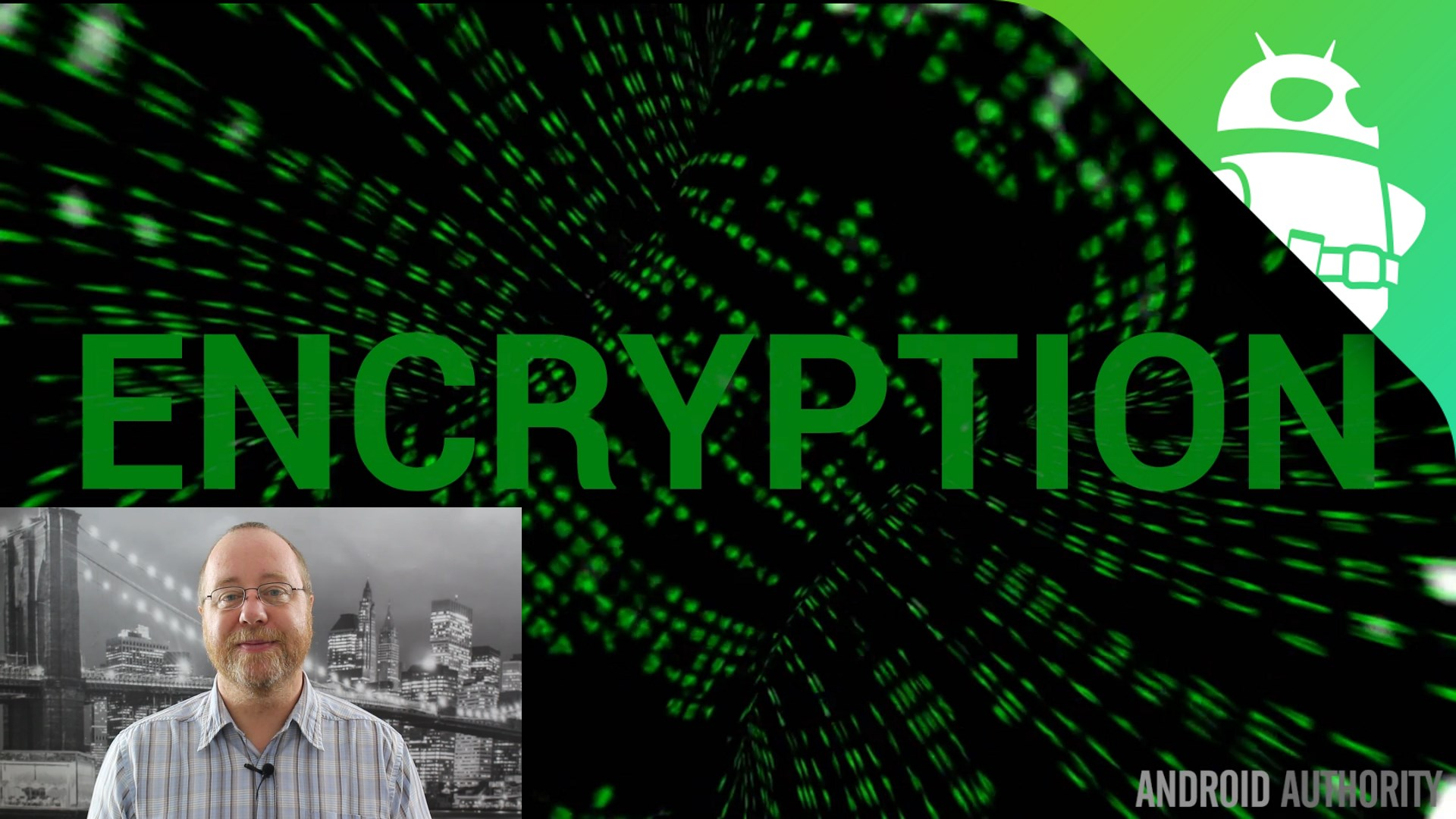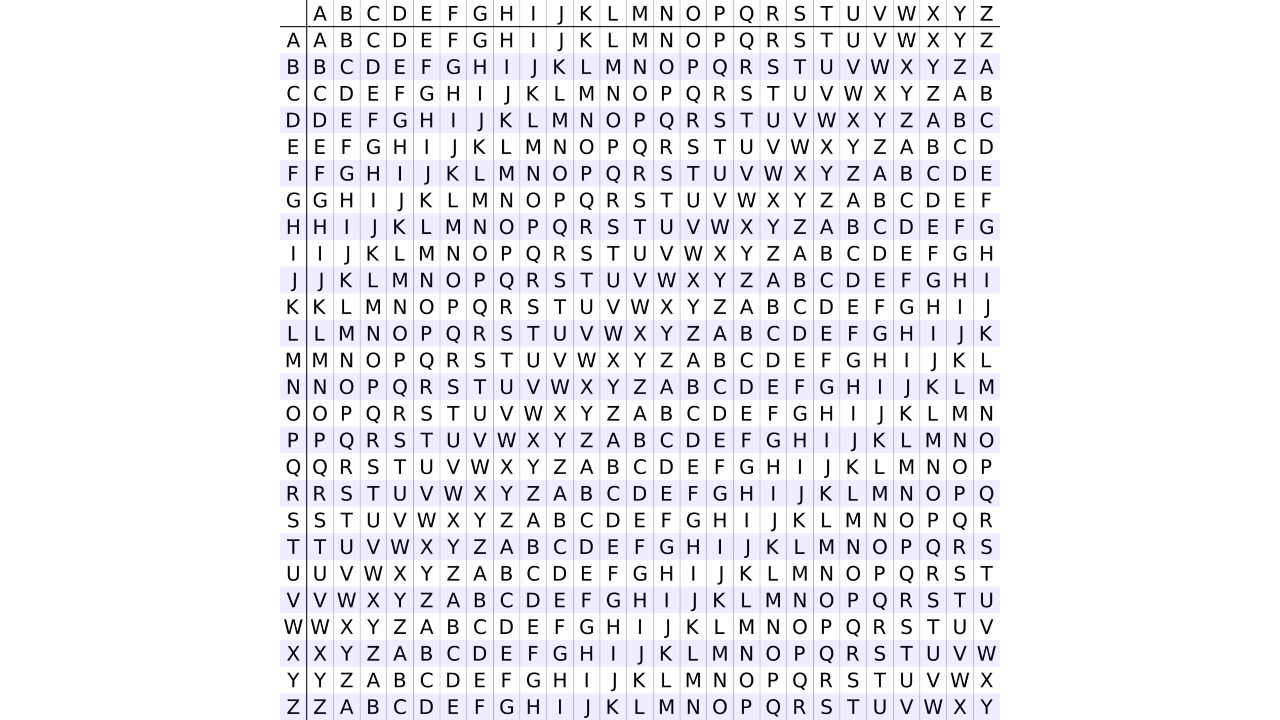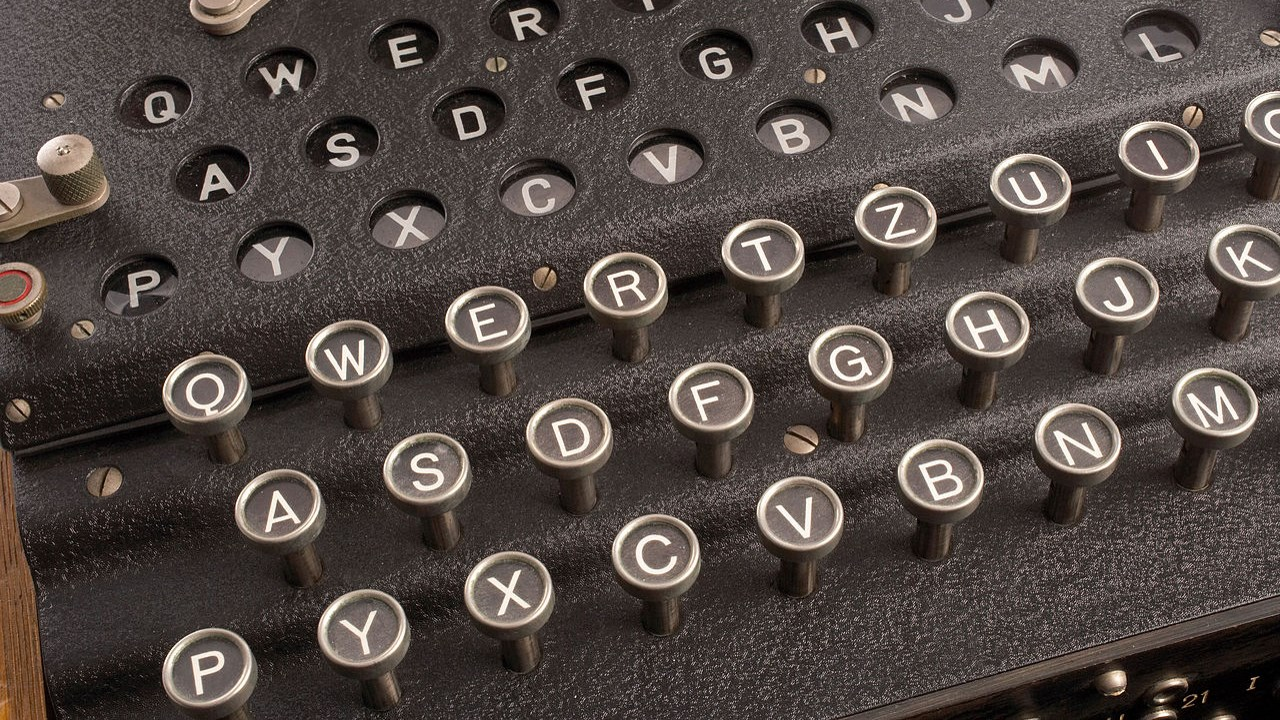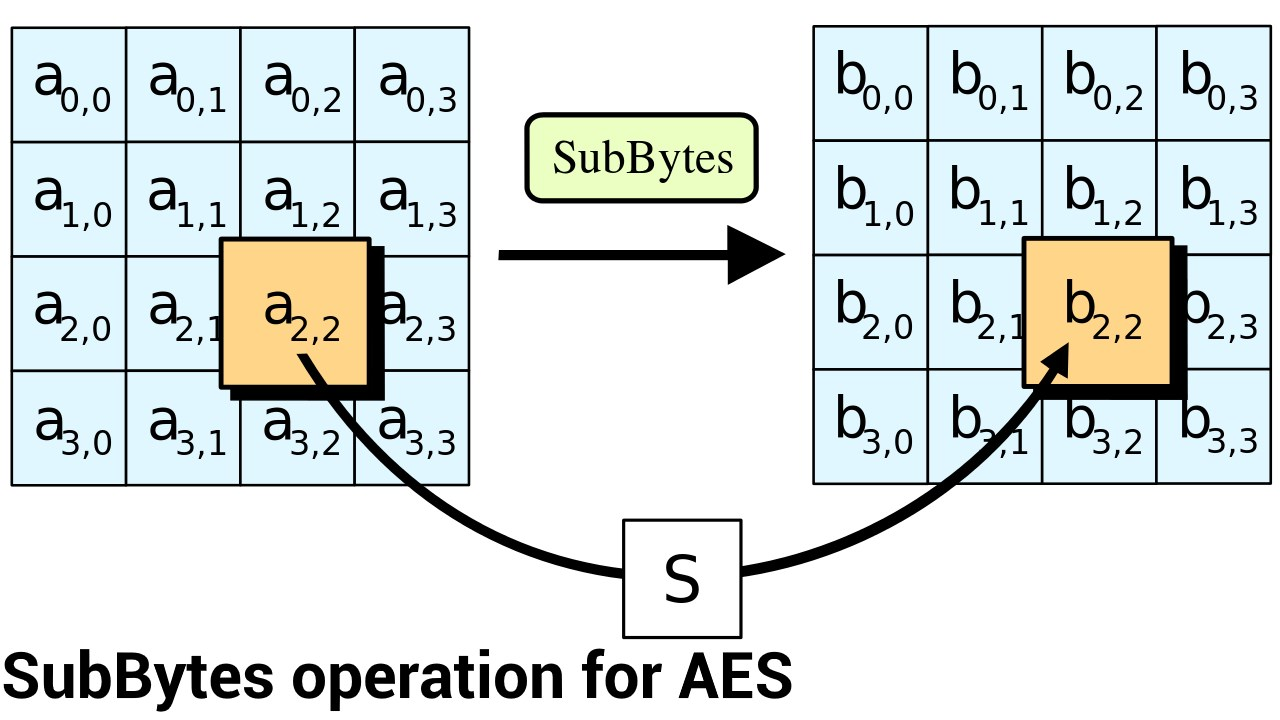Best daily deals

# How does encryption work? - Gary explains

You probably use some form of encryption almost every day, and you probably don't even think about it. But what is it and how does it work?
By
September 13, 2016You probably use encryption, in one form or another, every day. You might not know that you are, but you are. And my guess is that you don’t give it a second thought. Do you have a subscription based cable or satellite TV service? Guess what, some of that content will be encrypted. Do you connect to websites using https://? That’s more encryption. Ever created a .zip file with a password? You got it, that uses encryption.

I could go on and a list dozens of other examples of every day encryption, but I won’t. As for Android, it also supports encryption, not only for the web with https:// but also for your files and data. Android 6.0 Marshmallow used full disk encryption, while Android 7.0 Nougat has added the option for per-file encryption. The idea is that if your phone should fall into the hands of unfriendlies, then your private data is secure.

So what is encryption? It is the process of taking plain data, including text, and converting it into an unreadable (by humans or computers) form. The encryption process is based on a key, the analogy here being a lock which needs a key, and only people with the key can unlock (decrypt) the data and put it back into its original form. This means that anyone who gets hold of your encrypted data can’t read it unless they have the key.

As the Tom Jericho character in the excellent film Enigma put it, “It turns plain-text messages into gobbledygook. At the other end is another machine, which translates the message back to the original text.” Encryption and decryption!

## It all started with CaesarThe art of secret writing, what we would call encryption, has been around for at least 2500 years, however the most famous example from antiquity is that of the substitution cipher used by Julius Caesar to send messages to Cicero. A substitution cipher works like this, you start with the alphabet on one line and then add a second line with the alphabet shifted along a bit:

Code
``````A B C D E F G H I J K L M N O P Q R S T U V W X Y Z
X Y Z A B C D E F G H I J K L M N O P Q R S T U V W``````

If you want to encrypt the word “HELLO” then you take the first letter, H, and look at the letter below it, that gives you E. Then the E gives B and so on. The encrypted form of HELLO is EBIIL. To decrypt it you lookup E on the bottom row and see the H above it, then the B on the bottom to get the E above it and so on. Complete the process to get HELLO.

In this case the “key” is 3, because the alphabet has been shifted three to the right (you can also shift to the left instead). If you change to key to say 5, then you get this:

Code
``````A B C D E F G H I J K L M N O P Q R S T U V W X Y Z
V W X Y Z A B C D E F G H I J K L M N O P Q R S T U``````

Now the encrypted version of HELLO would be CZGGJ. Very different to EBIIL. In this case the key is 5. Magic!

However there are some major problems with this form of encryption. First of all there are only 26 keys. You might have heard of people talking about 128-bit keys or 256-bit keys, well this is a 5 bit key (i.e. 26 in binary is 11010). So it wouldn’t take too long to try all 26 variations and see which one starts to produce understandable text.

Secondly, English (and other languages) has certain characteristics. For example, E is the most popular letter in English, so if you had a good chunk of text you could see which letter appears the most frequently and then guess that it is E. Shift the bottom alphabet to match E with the most common character and you have probably cracked the code. Also there are only a few letters that can double up in English, like OO, LL, SS, EE and so on. Whenever you see a double like the II or GG (from the examples above) then you should try matching those on the alphabets first.

The combination of the small key and the fact that the same letter always encrypts to the same corresponding letter on the cipher alphabet means that this is very weak encryption. And today with computers doing the hard work, this is beyond weak!

## More alphabets and unbreakable encryption

The weaknesses of the Caesar substitution cipher can be slightly alleviated by using more than one shifted alphabet. The example below can be expanded to 26 shifted alphabets of which several are used at once, but not all of them.

Code
``````A B C D E F G H I J K L M N O P Q R S T U V W X Y Z
Z A B C D E F G H I J K L M N O P Q R S T U V W X Y
Y Z A B C D E F G H I J K L M N O P Q R S T U V W X
X Y Z A B C D E F G H I J K L M N O P Q R S T U V W
W X Y Z A B C D E F G H I J K L M N O P Q R S T U V
V W X Y Z A B C D E F G H I J K L M N O P Q R S T U``````

So if we set the key to WVY that means we use the alphabet starting with W first, then the one starting with V and finally the one starting with Y. This is then repeated to encode the entire message. So HELLO would become DZJHJ. Notice that now the double L in HELLO isn’t encoded as the same character, it is now J and then H. Also, the first J in the encrypted text is the code for L while the second on is the code for O. So J now doesn’t always represent the same plain text letter.

A version of this idea, with 26 alphabets, is the basis of the Vigenère cipher which was published in the 16th century by Blaise de Vigenère. A similar idea was also described by Giovan Battista Bellaso in 1553. The Vigenère cipher remained unbreakable for 300 years until it was cracked by Charles Babbage and then by Friedrich Kasiski. The secret to breaking the Vigenère cipher is understanding that ultimately the same words can be encoded using the same letters because the same alphabets are used again and again. So the word “AND” might be encoded different the first few times it appears, but ultimately it will be encoded using the same letters again. Repetition is generally the downfall of a cipher.Repetition is the weakness in the Caesar cipher, the Vigenère and all the variants, but there is one way to use an alphabet cipher to create an unbreakable secret code without repetitions, it is called the one-time pad. The idea is that rather than using a shifted alphabet then a random sequence of letters are used. This sequence must be truly random and must be the same length as the message.

Code
``````I S T H I S U N B R E A K A B L E
P S O V Y V U B M W S P A H Q T D``````

Rather than doing a straight substitution this time we use addition, with a twist. Each letter of the alphabet is assigned a number, A is 0, B is 1, C is 2 and so on. I is the 9th letter of the alphabet, which means it has a value of 8. P (the letter below it on our one-time-cipher pad) 15. 8 + 15 = 25 which means X. The second letter of our message is S, which has the value 18. It just so happens that S is also the letter on our one-time pad (which isn’t an issue at all). 18 + 18 = 36. Now here is the twist, there is no 36th letter of the alphabet. So we perform what is called a modulus operation. What that basically means is that we divided the result by 26 (the number of letters in the alphabet) and use the remainder. 36 / 26 = 1 remainder 10. The letter with the value of 10 is K. If you continue doing this the final encrypted message is:

Code
``X K H C G N O O N N W P K H R E H``

The reason this code is unbreakable is that you only ever use the key (the random string) once. This means that anyone trying to decode the message has no reference point and there is no repetition. The next message to be sent will use a completely different random key and so on.

The biggest problem with one-time pads, is getting the keys to the other party so that they can decrypt the message. Traditionally this was done using a book in the form of a notepad, with the different codes on each page. Which pages were in use would change every day and once a code was used it could be ripped from the pad and discarded. However these pads need to be transported in a secure method. Because if someone else gets the codes then the encryption can be cracked. This basically meant you needed to meet with the other party before hand and agree on which codes would be used and when. It is the most secure method but it is also the most cumbersome, and it certainly isn’t a workable solution for today’s modern digital world.

## The digital ageDuring the 20th century encryption became mechanized, the most famous example being the Enigma machine used by the Nazi’s during World War II. However after the war encryption became computerized. There are three big benefits to computerized cryptography:

1. Computers are flexible, unlike mechanical boxes, computers can be programmed to perform lots of different operations on a message and the number and complexity of these operations can be altered relatively quickly.
2. Speed.
3. Computers deal with binary numbers not just letters.

Points 1 and 2 are very important, especially when comparing computers to mechanical encryption methods. However the paradigm change is that computers deal with numbers and not letters. This means that encryption can be applied to any type of data. A text message, a picture, an audio file, a movie, a database, files on a smartphone and so on.

With the move from letters to binary came a change in how encryption is performed. Whole letters no longer need to be encrypted but instead the ones and zeros can be manipulated to yield new sequences. The word HELLO in 8-bit ASCII is 0100100001000101010011000100110001001111. From here the binary can be manipulated in a myriad of different ways. It can be split, shifted, added, multiplied, whatever.

The method used to process the ones and zeros is known as a cryptographic algorithm and there are many different types of algorithms. The main characteristics of an encryption algorithm are its security (can it be cracked) and its performance (how long does it take to encode or decode data).

Very broadly speaking there are two main types of digital encryption ciphers, stream ciphers and block ciphers. With a stream cipher the data is encrypted by byte at a time. The data is processed from the beginning to the end and is streamed through the encryption algorithm. RC4 is a famous example of a stream cipher. It was used in WEP and was an optional encryption method for several other protocols and products.

Stream ciphers are like one-time pads in that the data isn’t just encrypted against a single key, but rather a sequence of pseudo-random numbers which is based on the key. The difference between a one-time pad and a stream cipher is that with a one-time pad the key must be truly random. With stream ciphers using the same key means you get the same sequence of numbers, that is what makes it possible to decode the message. However without the key the sequence looks random and is therefore hard to break.

The weakness of RC4 was that under some circumstances and under some conditions (mainly when the same data was repeatedly encrypted) then it is possible to guess which numbers might comes next in the sequence. This guess reduces the number of possible combinations and allows a brute force attack (where every combination is tried) to be used. To get the attack to work lots of data is needed. The RC4 NO MORE attack needs to collect 75 hours worth of encrypted data, based on 4450 requests per seconds.

The other major type of cipher is the block cipher. This works by dividing the data into more manageable blocks, say 64-bit. Each block is processed several times, known as rounds (like in boxing). For each round the block is split into two equal parts, the left and the right. The right part remains untouched while the left part is encrypted using a special function, called a round function. The round function takes two inputs, the key and the right part (the part that went untouched). The result from the round function is then “added” to the left part using XOR.

This model is known as a Feistel Cipher, named after its inventor Horst Feistel who worked on encryption at IBM. His work ultimately led to the development of the Data Encryption Standard (DES). In 1977 DES became the official encryption standard for the United States and saw worldwide adoption. DES uses 16 rounds working on 64-bit blocks. The problem with DES is that the NSA limited the key size to 56-bits. While in 1977 this was sufficient, by the late 1990s it became possible for non-governmental organizations to break DES encrypted messages.

JARGON BUSTER
Exclusive OR (XOR) – This is a bit level logical operation that is applied to 2 input bits A and B. The Exclusive OR returns true or false (1 or 0) to the question, “A or B, but not, A and B”. You can think of it as, “one or the other but not both”. So, if A is 1 and B is 0 then that is one or the other, so the result is 1 (true). The same result applies to A is 0 and B is 1. But if A is 0 and B is 0 then the result is 0 (false), as both have the same value. False is also given for A is 1 and B is 1.

But the real magic of XOR is that it is reversible. If A XOR B = C then B XOR C = A, and A XOR C = B. This is very important for encryption as it means that data can be encrypted (where A is the data) using a key (B) to get the encrypted data (C). Later the encrypted data can be decrypted by XOR it with the key again to get the original data. The reason XOR is used in conjunction with complicated round functions and bit shifting operations is because on its own XOR can be broken using frequency analysis (because of the constantly repeating key).

In response to the weaknesses of DES, a new standard was proposed called Triple DES (3DES). Which basically encrypted the data three times with DES, but with three different keys. 3DES offered a way to increase the key size from 56-bits to 168-bits without the need to design a completely new block cipher algorithm. Due to some technical details, the effective key is 112 bits, but if you had a machine that could crack DES in 1 minute, it would take the same machine about 260,658 years to crack a Triple-DES key.

While DES had served its purpose for almost 25 years, the limited key length meant that it was time for another encryption standard. In 2001, the U.S. National Institute of Standards and Technology (NIST) published the Advanced Encryption Standard (AES). It isn’t a Feistel cipher, but rather a substitution-permutation network. It still uses blocks and rounds just like DES, however during each round the order of the bits in the block are swapped around and the result is combined with the key using XOR.

AES uses 128, 192 or 256 bits keys and works on blocks of 128-bits. The number of rounds used depends on the key size. The minimum is 10, which is used for 128-bit keys and the maximum is 14, which is used for 256-bit keys.## AES, Android and the ARMv8 architecture

AES is at the heart of the encryption subsystems in Android. For Android 5.0 and Android 6.0, Google mandated the use of AES with at least a 128-bit key for devices supporting full disk encryption. With Android 7, Google has moved over to file based encryption (FBE) which allows different files to be encrypted with different keys while allowing files to be decrypted independently. It looks like FBE in Android 7 uses 256-bit AES.

When ARM made the move from 32-bit to 64-bit it defined a new revision of its instruction set architecture called ARMv8. As well as defining the instruction set for 64-bit ARM chips, it also added new instructions to implement parts of the AES algorithm in hardware. During each round various bits are swapped around and substituted. How the bits are manipulated is well defined (and part of the standard) so the AES extensions in ARMv8 allow those parts of the encryption to happen in hardware rather than software.

The result is lightning fast encryption, which should have a negligible impact on overall system performance. The AOSP implementation of file-based encryption uses AES-256 and requires a performance of at least 50MB/s.

## Public key cryptography and wrap-up

Most of what we have discussed until now is known as symmetric encryption. To encrypt and decrypt a message both the sender and the recipient need to know the secret key. There is a form of encryption called asymmetric encryption where there are two keys, one for encrypting messages and a different one for decrypting them. The encryption key can be freely published for everyone who wants to send the recipient a message, however the decryption key needs to remain secret, but only needs to be known by the recipient. This means there is a public key and a private key. This system is the basis of how security on the Internet works, how the https:// protocol functions. However that is a story for another day!

In closing I want to add a caveat. Encryption is a complex topic and there is much more to encryption than I have written here.